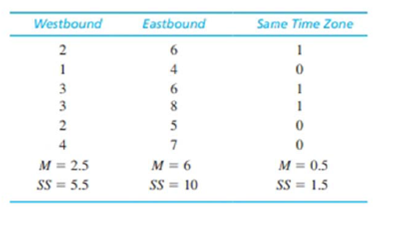Chapter 13, Problem 1REEssentials of Statistics for the B...

8th Edition
Frederick J Gravetter + 1 other
ISBN: 9781133956570

Solutions

Chapter
SectionEssentials of Statistics for the B...

8th Edition
Frederick J Gravetter + 1 other
ISBN: 9781133956570
Textbook Problem

A researcher examining the jet lag that people experience when flying long distances obtained the following data measuring the number of days required to adjust after a long flight. Do the data indicate significant differences in jet lag depending on the direction of travel? Test with α = .05.To determine
Whether there are significant mean difference between jetlag and the direction using α=0.05.

Explanation

Given info:

The data with appropriate values are provided in the question

 Westbound Eastbound Same time-zone N=18M=2.5SS=5.5 N=18M=6SS=10 N=18M=0.5SS=1.5 G=54∑X2=272

Calculation:

Step 1:

State the testing hypotheses:

Null hypothesis:

H0:μ1=μ2=μ3

Alternate hypothesis:

Ha: jetlag depends on the direction of travel.

Step 2: Compute the degrees of freedom:

dfbetween=k1=31=2

So,

dfwithin=5+5+5=15

With df=2,15 and α=0.05, CV from table is CV=3.68

Now,

SStotal=X2G2N=27254218=110

Similarly,

SSwithintreatment=SSinsideeachtreatment=5

Still sussing out bartleby?

Check out a sample textbook solution.

See a sample solution

The Solution to Your Study Problems

Bartleby provides explanations to thousands of textbook problems written by our experts, many with advanced degrees!

Get Started

find the real roots of each equation by factoring. 133. 14x2x+1=0

Applied Calculus for the Managerial, Life, and Social Sciences: A Brief Approach

Show that ddx1+tanhx1tanhx4=12ex/2.

Single Variable Calculus: Early Transcendentals, Volume I

The area of the shaded region is given by:

Study Guide for Stewart's Multivariable Calculus, 8th

Sometimes, Always, or Never: limxax=0.

Study Guide for Stewart's Single Variable Calculus: Early Transcendentals, 8th